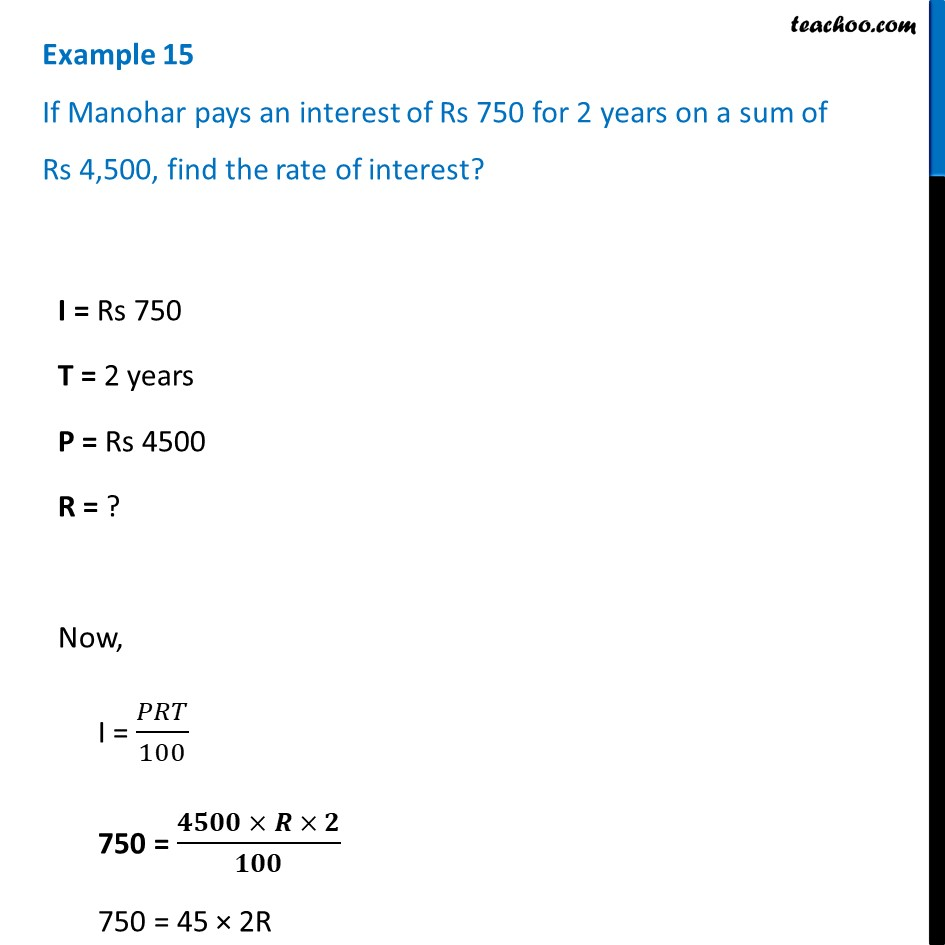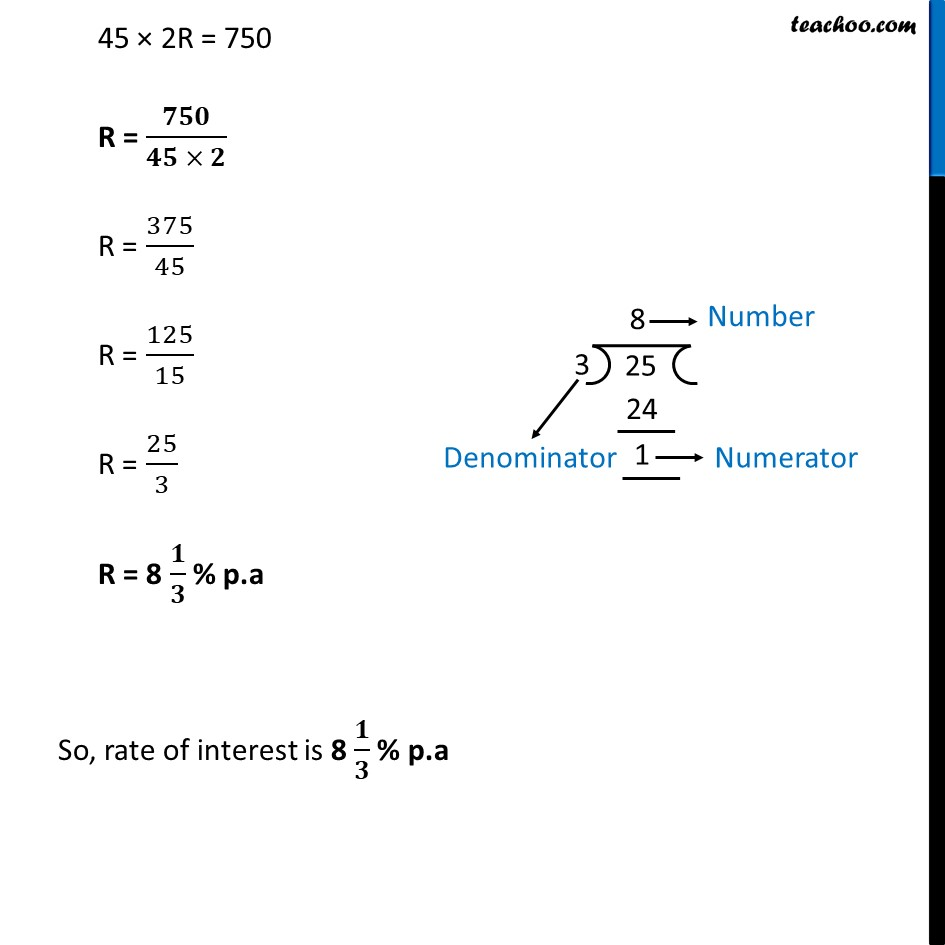Examples

Chapter 7 Class 7 Comparing Quantities
Serial order wiseLearn in your speed, with individual attention - Teachoo Maths 1-on-1 Class

### Transcript

Example 15 If Manohar pays an interest of Rs 750 for 2 years on a sum of Rs 4,500, find the rate of interest?I = Rs 750 T = 2 years P = Rs 4500 R = ? Now, I = 𝑃𝑅𝑇/100 750 = (𝟒𝟓𝟎𝟎 × 𝑹 × 𝟐)/𝟏𝟎𝟎 750 = 45 × 2R 45 × 2R = 750 R = 𝟕𝟓𝟎/(𝟒𝟓 × 𝟐) R = 375/45 R = 125/15 R = 25/3 R = 8 𝟏/𝟑 % p.a So, rate of interest is 8 𝟏/𝟑 % p.a